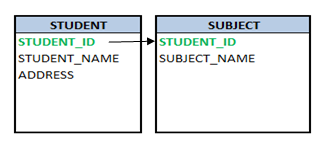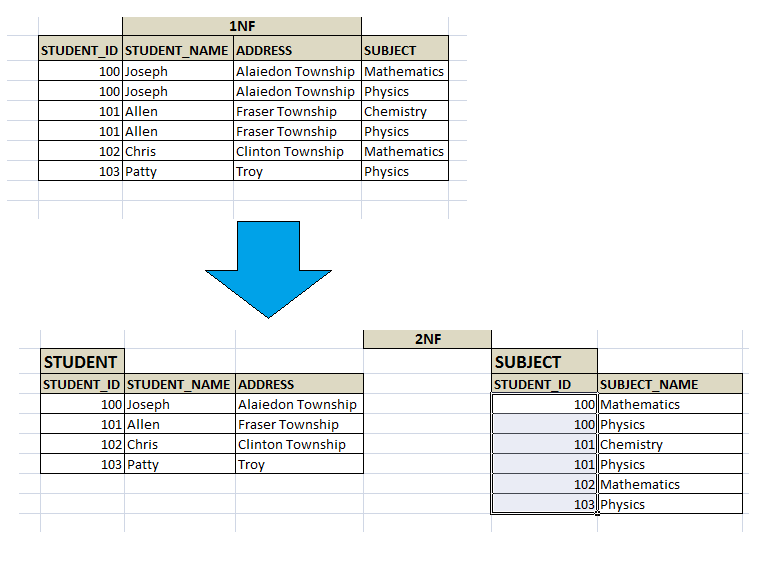# Second Normal Form (2NF)

## Second Normal Form (2NF)

A relation is said to be in a second normal form if and only if,

• it’s in first normal form
• Every non-key attributes are  identified by the use of primary key
• All subset of data, which applies to have multiple rows in a table must be removed and placed in a new table. And this new table and the parent table should be related by the use of foreign key.

In the 1NF STUDENT table above, Joseph and Allen have multiple rows because of their SUBJECTS.  Although it is in 1NF form, it wastes storage space by repeating whole of their information – name and address in each row. In addition, Student ID alone is strong enough to be a primary key. If we make Student_ID as primary, all other attributes in the table cannot be uniquely identified. This is because of multiple rows exists for single ID. Hence it does not satisfy second condition of 2NF.

So what we can do here is, apply the third condition of 2NF. Remove Subject from the STUDENT table and create a separate table for it. So the two tables are – STUDENT and SUBJECT. Now the STUDENT table will have only STUDENT information – STUDENT_ID, STUDENT_NAME and ADDRESS. New SUBJECT table will have STUDENT_ID and SUBJECT_NAME.Now there is no repeating group of columns in STUDENT table and STUDENT_ID is the primary key of STUDENT table. It uniquely identifies the Student name and address which are non key attributes of this table.  Hence it satisfies both 1NF and 2NF.

In the new SUBJECT table, Subject names opted by each student. Since same student cannot opt for same subject multiple times in academic year, there will not be any duplicity of data. But Student_ID alone is not unique; hence it cannot be a primary key. Both Student_ID and Subject_Name is unique in this table. Hence both of them together become a primary key. Hence SUBJECT table satisfies 1NF.

There is no non-key attributes in SUBJECT table. Hence we cannot verify second condition of 2NF. According to the third condition of 2NF, we have removed the data which is forming multiple rows and put those details in new table. It also states that there should be relationship between the original table and new table by using foreign key constraint.  In the SUBJECT table, STUDENT_ID is derived from STUDENT table. In this table, STUDENT _ID is part of primary key as well as it is a foreign key. Hence we can easily relate both STUDENT and SUBJECT table by using STUDENT_ID. Hence satisfies the third condition of 2NF.If we want to know which all subjects Joseph has opted for, we would query as below:

```SELECT std.STUDENT_ID,
std.STUDENT_NAME,
sb.SUBJECT
FROM STUDENT std, SUBJECT sb
WHERE std.STUDENT_ID = sb.STUDENT_ID
AND std.STUDENT_NAME = 'Joseph';```
Translate »